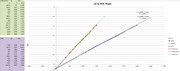# Mapping a slope formula

I have a custom made sensor for PPM/uS conductivity (amount of salt) of water.Using excel and a scatter plot and stumbled into a way for it to show the formula for the slope. y=.0008x - .2054
The formula is pretty darn close to what I'm actually plotting. good enough for government work anyway.

My problem is my math skills are terrible and I forgot my highschool algebra.... currently the X access in my chart is the reading I want to display in my application. Y access is the VDC I get from my voltmeter.
I need to solve for X when I have Y.

But that doesnt end my issue.
I know with an ADC I need to then map to a 0-1023 range.

The sensor has a range of 0-5000uS. Its accurate from 800uS-3000uS, or rather its less accurate outside that range.
My measurements will always be between 1000uS and 2800uS.

Whats the best way to do this mapping? Thanks for the input.

ok Well I think I got the reverse formula. x == 1027/4 + 1250 y
but still wondering what the best way to use the ADC.

y=.0008x - .2054

.2054 +y = .0008x

(.2054 +y) / .0008 = x

But it really doesnt matter when we're doing this through the Arduino (or other stuff). As you state the measurment is linear, when you have a circuit that yields a voltage you only need to see what value the Arduino analogRead reports at two known salt concentrations - the rest is linear interpolation which the map() function does!

ie, you do a small program with `Serial.println( analogRead(1) ) ;` and note the value for your two known values (these have to be integers, but if they are 0.05 you just use 500 then you know that you divide the reported integer value by 10000 to get the float value).

Say the Arduino say 315 and 873 for the saline values 0.05 and 1.2 (I have no idea if these values are reasonable - I'm just making some up)
Then write the real program where the output is
`Serial.println( map(analogRead(1), 315, 873, 500, 12000 )/10000.0 ) ;`this will then correctly linearily scale even when your input is below 315 or above 873.

Now we just need to get your circuit to yield a "reasonable" voltage to put into the Arduino. Remember the Aref pin voltage defines what 1023 is.

excellent reply thank you. I dont have anything in aref so I think that makes it 5v. Ill plug this sensor back up to the arduino, do some testing and report back.

Determine the slope of a line, which contains the points A (-1, -2), B (-2, 1):
Solution:
Here, x1 = -1, x2 = -2, y1 = -2, y2 = 1.
Slope of the line, m = [ (y2 - y1)/(x2 - x1)]
= [(1 + 2)/(-2 + 1)]
= [3/(-1)]
Slope of the line (m) = -3
The slope formula of the line is:
Slope (m) = [ (y2 - y1)/(x2 - x1)]
Slope (m) =Rise / Run
(Rise = change in the y co-ordinate; Run = change in the x co-ordinate)
In the above given graph, the Rise is given for the points B (4,2) and C (4,4) and the Run is given for the points A (2,2) and B (4,2)
Rise = change in the y - coordinate = 4-2 =2
Run = change in the x- coordinate = 4-2 =2
Using the slope formula, we get :
Slope( m ) = Rise / Run = 2 / 2 =1

Serial.println( map(analogRead(1), 315, 873, 500, 12000 )/10000.0 ) ;

Be aware that the map function will also 'map' outside the given scope of 500 - 12000 if analogRead() is below 315 or above 873.

If you do not want that use the constrain function : x = constrain (x, LOWER, UPPER);